TU Vienna logic.at Theory and Logic Group (E185-2)

## LK

Basically we use Gentzen's version of LK (see ), but extend it by equality and definition rules. The axioms may be arbitrary atomic sequents. There are two groups of rules, the logical and the structural ones. All rules with the exception of cut have left and right versions; left versions are denoted by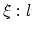, right versions by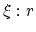. Every logical rule introduces a logical operator on the left or on the right side of a sequent. Structural rules serve the purpose of making logical inferences possible (e.g. permutation) or to put proofs together (cut).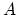and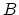denote formulas,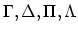sequences of formulas. In the rules there are introducing or auxiliary formulas (in the premises) and introduced or principal formulas in the conclusion. We indicate these formulas for all rules. In particular we mark the auxiliary formulas by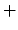and the principal ones by.

The logical rules:

•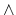-introduction: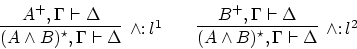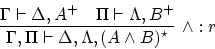•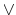-introduction: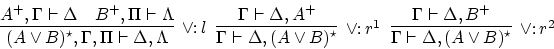•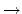-introduction: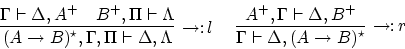•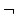-introduction: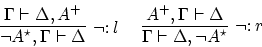•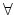-introduction: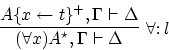where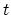is an arbitrary term containing only free variables.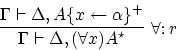where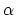is a free variable which may not occur in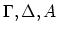.is called an eigenvariable.
• The logical rules for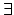-introduction (the variable conditions for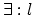are these for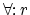, and similarly for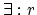and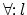):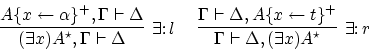The structural rules:

• permutation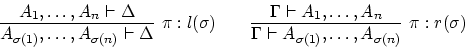where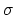is a permutation. In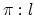all formulas on the left side of the conclusion sequent are principal formulas and all formulas on the left side of the premise sequent are auxiliary formulas; similarly for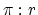.
• weakening: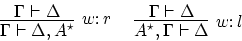• contraction: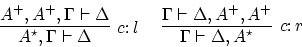• The cut rule: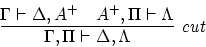As unary structural rules are not important in classical logic we frequently indicate their use by double lines, e.g.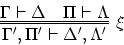indicates the application of the rule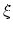plus a sequence of unary structural rules before and/or after the application of.